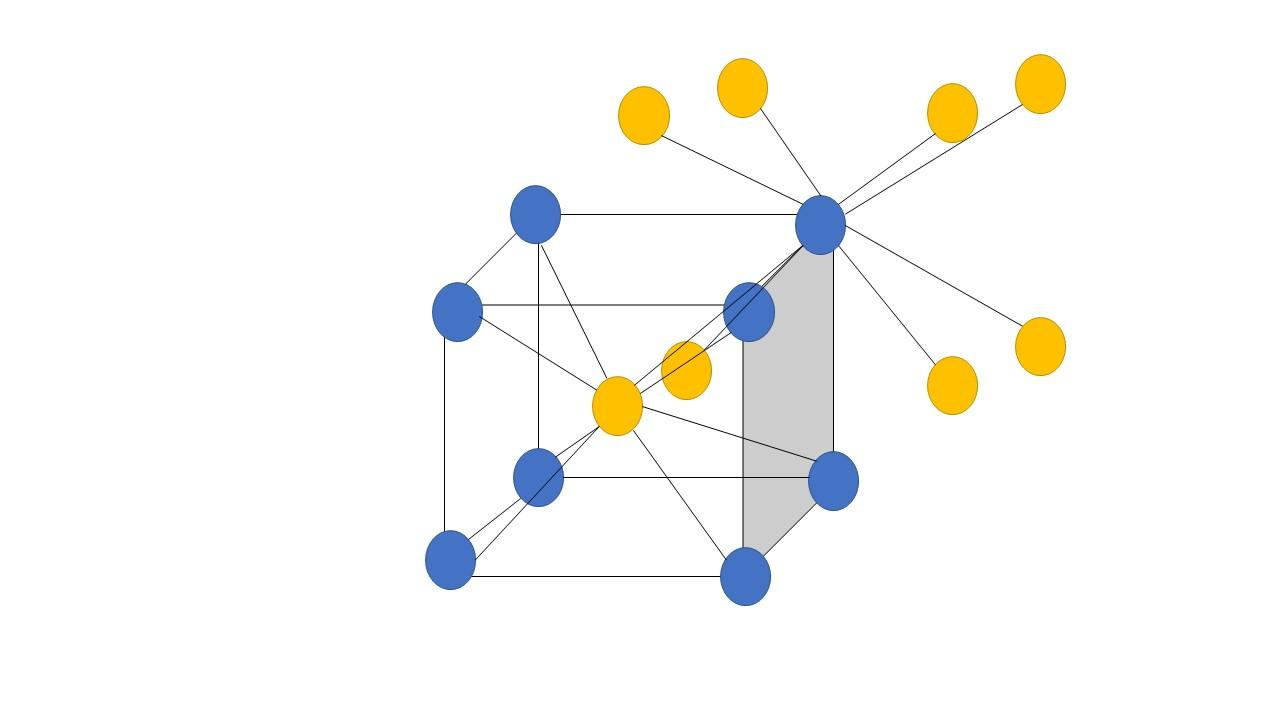QuestionAnswers

# Coordination number of $C{s^ + }$ and $C{l^ - }$ in $CsCl$ crystal are: A. $8,8$B. $4,4$ C. $6,6$D. $8,4$Hint: All crystal lattices are built up of repeating unit cells, and in a unit cell, an atom's coordination number is referred to the number of atoms it is touching or in direct contact with or the number of ions that surrounds an ion of the opposite charge within a crystal lattice. For example: In the bcc lattice the coordination number of the corner atoms is eight and the coordination number of the body-center atom is also eight.

Before calculating the coordination number of $CsCl$ lattice first we need to know the type of unit cell it has and the cesium ions in $CsCl$ forms the simple cubic arrangement and the chloride ions occupy the interstitial sites or is present at the center of the lattice, It can be vice-versa as well that the cesium ions occupy the center of the lattice and the chloride ions show the simple cubic arrangement.
Hence, the center ion is the $C{s^ + }$ ion which is surrounded or directly touching the eight $C{l^ - }$ ions present at the corners of the cube. Similarly, each $C{l^ - }$ ion present at the corners is also surrounded by or directly touching to the eight $C{s^ + }$ ions present at the center of the surrounding unit cells. Thus, the coordination numbers in this type of crystal are both $8$.  This can be easily explained with the help of a diagram as follows:Where the yellow atoms are the ones that are present at the body center which is connected to eight blue atoms or the corner atoms, similarly the blue atoms or the corner atoms are connected to eight yellow atoms or the body center atoms.

Therefore, the correct answer is $8,8$ (Option A).

Note:A student may confuse about that why $CsCl$ is not a body-centered lattice as one of the ions sits at the body center and the other at the corner well this is because for a lattice to be body-centered it requires the same ion to occupy the corners and the center of the lattice.

Neural Control and CoordinationThe Solid StateClassification of Elements and Periodicity in PropertiesDerivation of Reynolds NumberNCERT Book for Class 12 Chemistry PDF in HindiNumber of Moles FormulaCBSE Class 12 Physics Dual Nature of Radiation and Matter FormulaSolid State PhysicsUses of Boron and AluminiumCBSE Class 9 Maths Chapter 1 - Number Systems FormulasImportant Questions for CBSE Class 12 Chemistry Chapter 1 - The Solid StateImportant Questions for CBSE Class 12 Chemistry Chapter 9 - Coordination CompoundsNCERT Books Free Download for Class 12 Chemistry Chapter 1 - The Solid StateImportant Questions for CBSE Class 12 Chemistry Chapter 16 - Chemistry in Everyday lifeImportant Questions for CBSE Class 12 Chemistry Chapter 6 - General Principles and Processes of Isolation of ElementsImportant Questions for CBSE Class 12 ChemistryNCERT Books Free Download for Class 12 Chemistry Chapter 9 - Coordination CompoundsImportant Questions for CBSE Class 12 Chemistry Chapter 5 - Surface ChemistryImportant Questions for CBSE Class 11 Chemistry Chapter 12 - Organic Chemistry - Some Basic Principles and TechniquesImportant Questions for CBSE Class 12 Chemistry Chapter 12 - Aldehydes, Ketones and Carboxylic AcidsChemistry Question Paper for CBSE Class 12CBSE Class 12 Chemistry Question Paper 2020Chemistry Question Paper for CBSE Class 12 - 2013Chemistry Question Paper for CBSE Class 12 - 2015CBSE Class 12 Chemistry Question Paper 2019 - Free PDFCBSE Class 12 Chemistry Question Paper 2017 - Free PDFCBSE Class 12 Chemistry Question Paper 2018 - Free PDFPrevious Year Question Paper for CBSE Class 12 Chemistry - 2014Chemistry Question Paper for CBSE Class 12 - 2016 Set 1 EChemistry Question Paper for CBSE Class 12 - 2016 Set 1 SNCERT Solutions for Class 12 Chemistry Chapter 9NCERT Solutions for Class 12 Chemistry Chapter 1 The Solid State in HindiNCERT Solutions for Class 12 Chemistry Chapter 1NCERT Solutions for Class 12 Chemistry Chapter 9 Coordination Compounds in HindiNCERT Solutions for Class 11 Biology Chapter 21NCERT Solutions for Class 11 Biology Chapter 22NCERT Solutions for Class 10 Science Chapter 7NCERT Solutions for Class 12 Chemistry Chapter 6 General Principles and Processes of Isolation of Elements in HindiNCERT Solutions for Class 12 Chemistry Chapter 5 Surface Chemistry in HindiNCERT Solutions for Class 11 Chemistry Chapter 12 Organic Chemistry Some Basic Principles and Techniques In Hindi